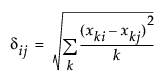Publication date: 11/29/2021

## Attributes List Format

When the data is an attributes list, it is converted to a distance matrix and then MDS is applied. The distance matrix is calculated using Euclidean distance. For each pair of items define the distance between the items by:where k is the number of attributes.

Note: For an advanced example of the MDS platform, see the San Francisco Crime Distances.jmp sample data table and the source script for that table. The script creates the distance matrix using pairwise correlations. The resulting distance matrix is then used to explore the relationships between crime categories.